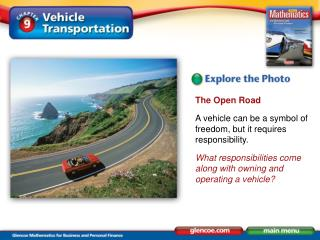DownloadDownload PresentationThe Open Road A vehicle can be a symbol of freedom, but it requires responsibility.

# The Open Road A vehicle can be a symbol of freedom, but it requires responsibility.

Télécharger la présentation## The Open Road A vehicle can be a symbol of freedom, but it requires responsibility.

- - - - - - - - - - - - - - - - - - - - - - - - - - - E N D - - - - - - - - - - - - - - - - - - - - - - - - - - -
##### Presentation Transcript

1. The Open Road A vehicle can be a symbol of freedom, but it requires responsibility. What responsibilities come along with owning and operating a vehicle?

2. Lesson Objective Compute the total cost per mile of operating and maintaining a vehicle. Content Vocabulary • variable costs variable costs Costs of producing a product that vary directly with the number of units produced, such as raw materials and packaging. Also, vehicle costs that increase the more you drive, such as gasoline and tires. fixed costs Production or vehicle costs which remain about the same regardless of how much you produce or drive, such as building rent or vehicle registration. depreciation The decrease in the value of your vehicle from one year to the next because of its age and condition. • fixed costs • depreciation

3. Example 1 Ann Jones purchased a used vehicle for \$4,000 one year ago. She drove 9,000 miles during the year and kept a record of all her expenses. She estimates the vehicle’s present value at \$3,200. Her fixed and variable costs are shown in the figure below. What was the cost per mile for Jones to operate her vehicle last year?

4. Example 1 (cont.)

5. Example 1 Answer Step: Find the cost per mile. (Annual Variable Cost + Annual Fixed Cost) ÷ Number of Miles Driven (\$819.20 + \$1,261.65) ÷ 9,000 = \$2,080.85 ÷ \$9,000= \$0.231 or \$0.23 per mile

6. Example 2 Lucas Perry purchased a new four-door car 2 years ago at a price of \$21,750. Kiplinger estimates it is worth \$13,920 today. The Complete Car Cost Guide computes the annual variable cost to be \$1,330.20 per year with insurance costing \$1,461 per year.

7. Example 2 (cont.) Perry paid \$112.60 for license and registration fees and drove 16,500 miles during the year. After computing the depreciation and the total annual cost, find the cost per mile.

8. Example 2 Answer: Step 1 Find the depreciation. Purchase Price – Today’s Worth Number of Years Owned (\$21,750.00 – \$13,920.00) ÷ 2 \$7,830.00 ÷ 2 = \$3,915.00

9. Example 2 Answer: Step 2 Find the total annual cost. \$1,330.20 + (\$1,461.00 + \$3,915.00 + \$112.60) = \$6,818.80

10. Example 2 Answer: Step 3 Find the cost per mile. (Annual Variable Cost + Annual Fixed Cost) ÷ Number of Miles Driven \$6,818.80 ÷ 16,500 = \$0.4132 or \$0.41 per mile

11. Practice 1 Kim Leung drove his SUV 11,500 miles. Fixed costs totaled \$3,343. Variable costs totaled \$1,511. What was the total annual cost? What was the cost per mile?

12. Practice 1 Answer Total annual cost: \$4,854 Cost per mile: \$0.42 per mile

13. Practice 2 Kim Misawa drove her vehicle 13,000 miles. Fixed costs totaled \$2,693. Variable costs totaled \$1,471. What was the total annual cost? What was the cost per mile?

14. Practice 2 Answer Total annual cost: \$4,164 Cost per mile: \$0.32 per mile